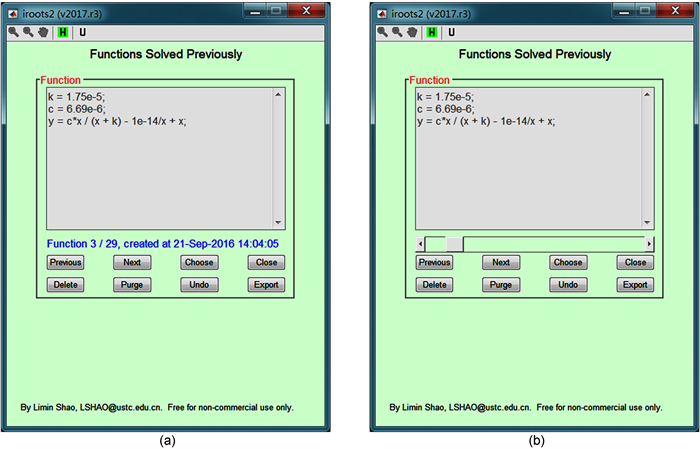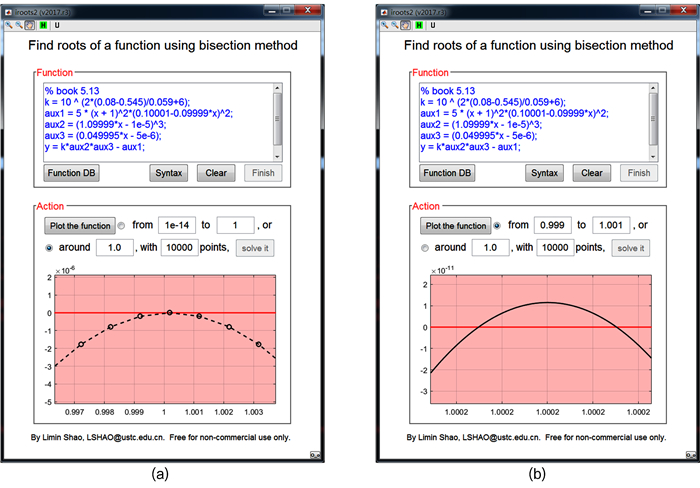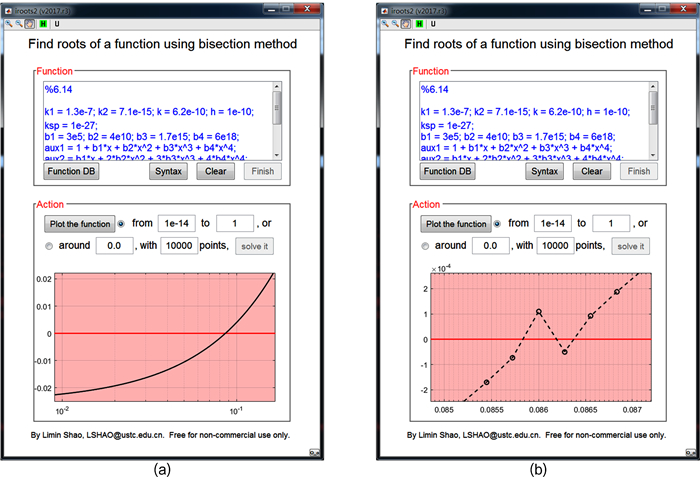## Developing a Software Package to Plot and Solve Complex Equations in Chemical Analysis

 基金资助: 安徽省重大教学研究项目.  2015zdjy006

 Fund supported: 安徽省重大教学研究项目.  2015zdjy006Abstract

Accurate analysis of chemical equilibria involves solving complex equations, which requires adequate knowledge on algorithms and programming languages. People who major in analytical chemistry often lack such knowledge. As a result, it is difficult to adopt accurate analysis in analytical chemistry on a large scale. To overcome such difficulty, we developed a software package with Matlab. The software solves complex equations efficiently through an intuitive interface, a graph, and natural interactions, whilst requires little programming on users. This paper introduced theory and features of the software package, and explained its usage in detail with 3 examples of complex chemical equilibria. An Android version was also developed and provided free of charge.

Keywords： Chemical equilibrium ; Rigorous solution ; Software development ; Matlab language ; Android system

SHAO Li-Min. Developing a Software Package to Plot and Solve Complex Equations in Chemical Analysis. University Chemistry[J], 2017, 32(10): 52-60 doi:10.3866/PKU.DXHX201705025

## 2 软件安装和首次运行

iroots2要求Matlab的最低版本是7.10.0.499 (R2010a)。如果Matlab版本太低，软件给出相应提示。

### 图1### 3.1 输入方程

1)变量区分大小写。

2)字母xy是指定的自变量和因变量，不能他用。

3)以%开始的行是注释。建议进行必要的注释，以备参考，因为所有用户输入的方程都被自动保存在一个数据库中。

4)如果方程表达式比较复杂，可以使用一些临时变量，参见“Syntax”按钮提供的实例。

5)每条语句应该以分号结束(用户如果忽略，软件会智能添加)。

6)运算符+、-、*、/、^分别代表加、减、乘、除、和乘方。对于开n次方，可以使用^(1/n)。负数开奇数次方Matlab给出复数结果，如(-8)^(1/3) = 1.0000 + 1.7321i，为了避免这一点，建议使用nthroot，如nthroot(-8, 3) = -2。

(7)如果表达式较长，输入时会自动折行。用户也可以自己断行，断行处添加省略号…。

### 图2(a)缺省界面；(b)鼠标右键单击蓝色文本后的界面

## 4 软件应用

const = 10 ^ (2 * (0.08 -0.545)/0.059 + 6);

aux1 = 5 * (x + 1)^2 * (0.10001 -0.09999 * x)^2;

aux2 = (1.09999 * x -1e-5)^3;

aux3 = (0.049995 * x -5e-6);

y = const * aux2 * aux3 -aux1;

### 图3(a)在1.0附近的图像；(b) 0.999－1.001上的图像

k1 = 1.3e-7; k2 = 7.1e-15; k = 6.2e-10; h = 1e-10; ksp = 1e-27;

b1 = 3e5; b2 = 4e10; b3 = 1.7e15; b4 = 6e18;

aux1 = 1 + b1 * x + b2 * x^2 + b3 * x^3 + b4 * x^4;

aux2 = b1 * x + 2 * b2 * x^2 + 3 * b3 * x^3 + 4 * b4 * x^4;

aux3 = k1 * k2 / (h^2 + k1 * h + k1 * k2);

aux4 = k / (h + k);

y = ksp * aux2 / (0.1 -x / aux4) / aux3 -(0.1 -x / aux4) * aux1 / aux2;

Cd2+-CN-配合物的生成致使[CN-] < 0.10；但是CdS非常难溶，释放出的Cd2+很少，故[CN-]也不会太小。这样，未知数[CN-]很有可能处于软件默认的求根区间10-14 -1之中，于是直接点击“Plot the function”进行绘图。

### 图4(a)放大图像；(b)交点附近的放大图像

$\left[{{{\rm{H}}^ + }} \right] + 2\frac{{{K_{{\rm{sp}}}}}}{{\left[{{{\rm{C}}_2}{\rm{O}}_4^{2-}} \right]}} = \frac{{{K_1}\left[{{{\rm{H}}^ + }} \right] + 2{K_1}{K_2}}}{{{{\left[{{{\rm{H}}^ + }} \right]}^2} + {K_1}\left[{{{\rm{H}}^ + }} \right] + {K_1}{K_2}}}\left( {0.043 + \frac{{{K_{{\rm{sp}}}}}}{{\left[{{{\rm{C}}_2}{\rm{O}}_4^{2-}} \right]}}} \right) + 0.014 + \frac{{{K_{\rm{w}}}}}{{\left[{{{\rm{H}}^ + }} \right]}}$

$\left[{{{\rm{C}}_2}{\rm{O}}_4^{2-}} \right] = \frac{{{K_1}{K_2}}}{{{{\left[{{{\rm{H}}^ + }} \right]}^2} + {K_1}\left[{{{\rm{H}}^ + }} \right] + {K_1}{K_2}}}\left( {0.043 + \frac{{{K_{{\rm{sp}}}}}}{{\left[{{{\rm{C}}_2}{\rm{O}}_4^{2-}} \right]}}} \right)$

ksp = 2.3e-9; k1 = 5.9e-2; k2 = 6.4e-5;

aux1 = x^2 + k1 * x + k1 * k2;

aux2 = (k1 * x + 2 * k1 * k2) * 0.043 / aux1 + 0.014 + 1e-14 / x -x;

aux3 = (2 * ksp -ksp * (k1 * x + 2 * k1 * k2)/aux1)/aux2;

y = aux3 -k1 * k2/aux1 * (0.043 + ksp/aux3);

### 图5(a)原图像；(b)放大图像

## 参考文献 原文顺序 文献年度倒序 文中引用次数倒序 被引期刊影响因子

/

 〈〉